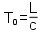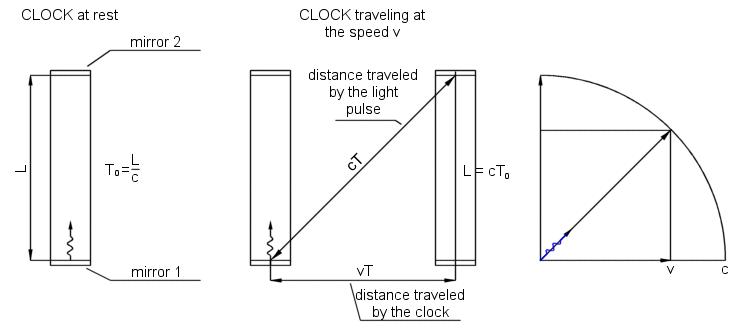# Time dilation

Time dilation

Consider a clock consisting of two mirrors 1 and 2, between which a light pulse is bouncing. The distance between mirrors is L and the clock ticks once each time the light pulse hits one of the mirrors. In the frame where the clock is at rest, the light pulse travels a distance of L and the period of the clock is L divided by the speed of lighttime dilation

When our clock moves at the speed v, travelling a distance of vT in a time T, light pulse direction is changed and light pulse travels a longer distance than the pulse in a clock at rest. Because the speed of light is constant, light pulse needs more time to reach second mirror.Thus, from Pythagorean theorem we can writeBecause the light pulse needs more time to reach second mirror, the clock counts slower.

In addition to the time dilation related to the motion (relative velocity) there is also gravitational time dilation. Gravitational time dilation is a consequence of special relativity in accelerated frames of reference. In general relativity, it is considered to be a difference in the passage of proper time at different positions as described by a metric tensor of spacetime.

Time dilation - Wikipedia

In physics, the twin paradox is a thought experiment in special relativity involving identical twins, one of the twins makes a space journey at a speed close to the speed of light and when returns home he find that the twin who stayed on Earth is older. This result appears puzzling because each twin sees the other twin as moving, and so, according to an incorrect naive application of time dilation and the principle of relativity, each should paradoxically find the other to have aged more slowly. However, this scenario can be resolved within the standard framework of special relativity: the travelling twin's trajectory involves two different inertial frames, one for the outbound journey and one for the inbound journey, and so there is no symmetry between the spacetime paths of the two twins. Therefore the twin paradox is not a paradox in the sense of a logical contradiction.

Has it been confirmed?

Yes, two scientists took four atomic clocks and set them to be at exactly the same time. They flew twice around the world, first eastward, then westward, and compared the clocks against others that remained at ground. When they got back, they found out that clocks disagree with one another, and their differences were consistent with the predictions of special and general relativity.

Clocks on the ISS space station run slightly slower than reference clocks on Earth, while clocks on GPS and Galileo satellites run slightly faster.

time, dilation, experiment, twin, paradox, experiment, Hafele-Keating experiment,

Hafele-Keating experiment - Wikipedia